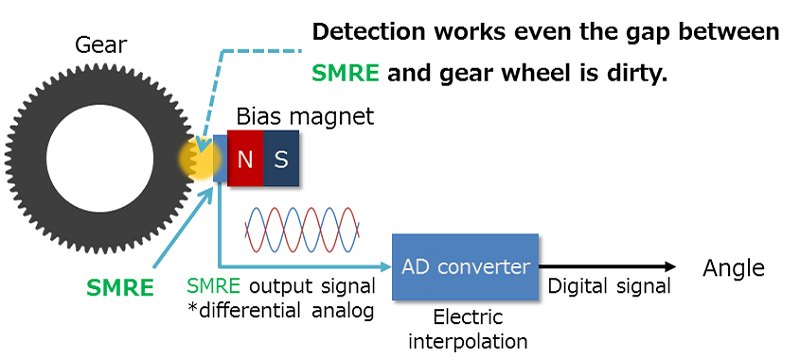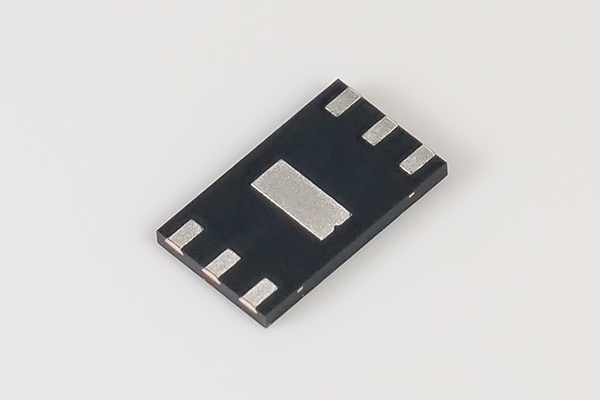# Semiconductor Magnetoresistive Elements (SMRE)

Suitable for high accuracy detection of gear rotation.

Since a Sn doped single crystal film InSb is used as a material for the sensing element, the element has excellent magnetoresistive temperature characteristics and very small neutral voltage temperature drift.
This element is mainly used for detection of gear rotation.
Semiconductor magnetoresistive element MS product family comprises the back bais holder built-in dual inline package (DIP) type with A/B,Za/Zb phase output as well as small out-line package (SON) type which can be set in thin/small place with A/B phase output.

Products

## A differential output semiconductor magnetoresistive element, (SMRE), for module, “m“ = 0.3 gear wheelsMS0031

The MS0031 eliminates in-phase noise with differential output signals of A+/A- and B+/B-.  The MS0031 achieves high resolution rotation angle detection exceeding 20-bit from electronic interpolation, achieving top-level performance among magnetoresistive elements*.  This is due to its stunning temperature characteristics while having extremely small variability among individual devices.

● A+/A-, B+/B- Differential OutputMS0031

Rotary encoder

• Machine tool spindle position and speed control
• Machining Machine tool precise rotation angle control
• Hoisting motor
• Electrical injection molding machine main shaft control
• CT scanner control
• X-ray transfer bed position control
• Motor control
• Crane slewing azimuth angle detection

Motor controlling

• Industrial Robots
• Machine Tools
• AC Servo motors

Linear encoder

• Linear control

## Lineup

`Part Number`
```Part
Status```
```Pitch
[mm]```
```Input / Output
Resistance (MIN.)
[Ω]```
```Input / Output
Resistance (TYP.)
[Ω]```
```Input / Output
Resistance (MAX.)
[Ω]```
`Number of Elements`
`Other`
```Operating
Temperature
[℃]```
```Device
Package```
```Number
of Pins```
`MS0020`
`MP`
`0.634`
`205`

`330`
`1`

`-40 to 100`
`SON`
`4`
`MS0030`
`MP`
`0.96`
`270`

`375`
`1`

`-40 to 125`
`SON`
`4`
`MS0031`
`MP`
`0.96`
`280`

`480`
`1`

`-40 to 125`
`SON`
`6`
`MS0041`
`MP`
`1.28`
`270`

`375`
`1`

`-40 to 125`
`SON`
`4`
`MS0042`
`MP`
`1.28`
`290`

`420`
`2`

`-40 to 125`
`SON`
`8`
`MS0043`
`MP`
`1.28`
`275`

`410`
`1`

`-40 to 125`
`SON`
`6`
`MS0050`
`MP`
`1.6`
`270`

`375`
`1`

`-40 to 125`
`SON`
`4`
`MS0060`
`MP`
`1.92`
`300`

`500`
`1`

`-40 to 125`
`SON`
`4`
`MS0082`
`MP`
`2.512`
`465`

`755`
`1`

`-40 to 125`
`SON`
`4`
`MS0083`
`MP`
`2.624`
`290`

`480`
`1`

`-40 to 125`
`SON`
`6`
`MSP042`
`MP`
`1.28`
`230`

`375`
`2`

`-40 to 100`
`DIP`
`8`
`MSP043`
`MP`
`1.28`
`230`

`375`
`2`

`-40 to 100`
`DIP`
`8`
`MSP082`
`MP`
`2.512`
`465`

`755`
`2`

`-40 to 100`
`DIP`
`8`
`MSP204`
`MP`
`6.84`
`290`

`420`
`4`

`-40 to 125`
`DIP`
`8`
`MSP254`
`MP`
`8.64`
`290`

`420`
`4`

`-40 to 125`
`DIP`
`8`